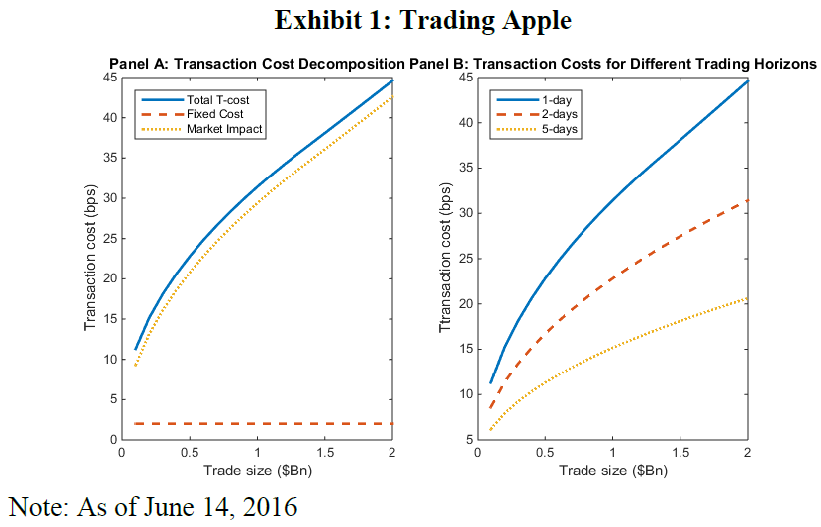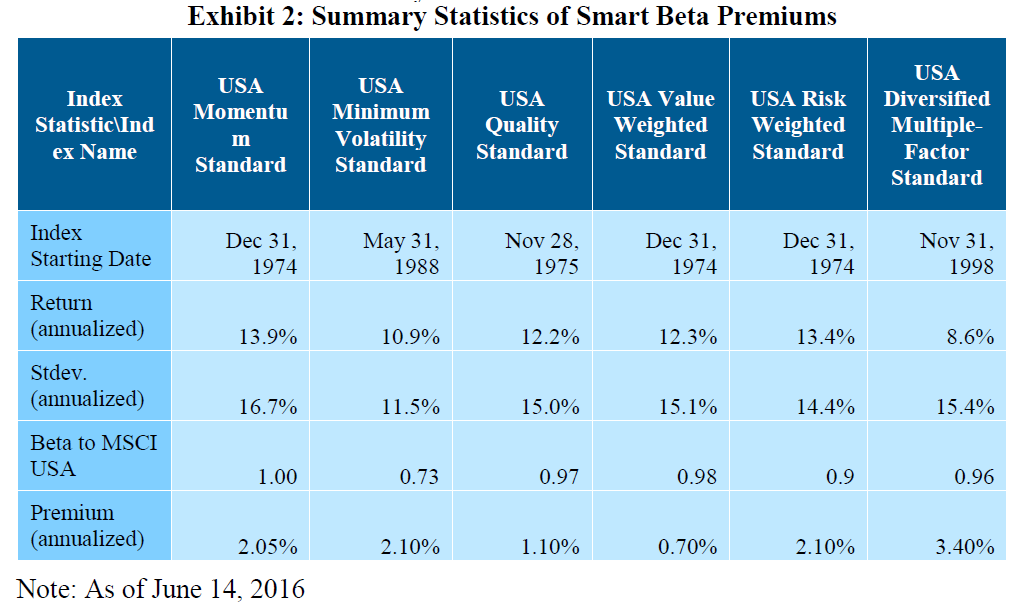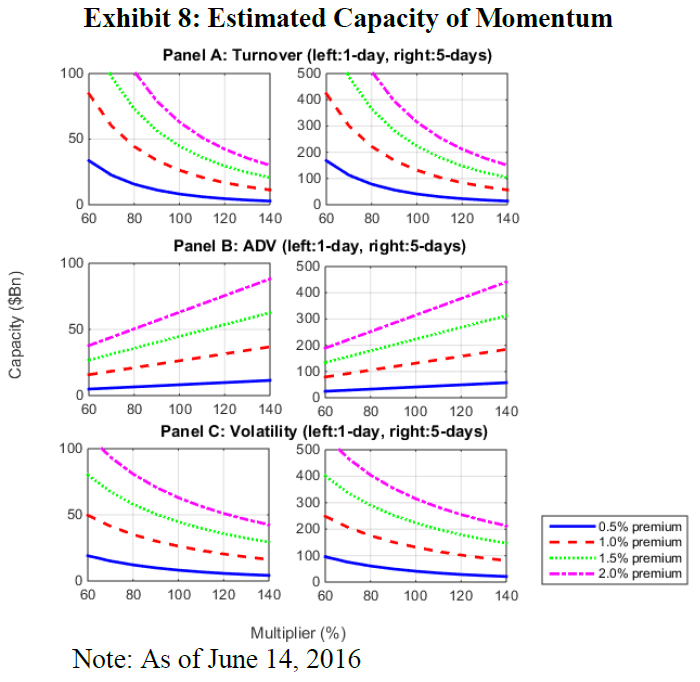ETFs and factor investing are on the tip of everyone’s tongue these days. Factor investing is being couched as a “new” thing, despite the fact that institutional investors have been deploying these strategies for years. (See this working paper discussing the effective use of smart beta strategies by institutional investors.)

However, because factor investing is now directly accessible via ETFs, those who are unfamiliar with factor investing are asking questions about how these “new” funds will affect the market.

Two burning questions many investors have:

• What is the overall capacity of smart beta funds?
• What is the capacity of momentum-based funds, specifically?

These are great questions and they have been examined in a few papers recently. There have been arguments on both sides of the aisle:

• Momentum can survive transaction costs — A new paper can be found here (by the AQR folks) and our summary can be found here.
• Transaction costs are large and (generally) subsume any (or most of the) premiums for the momentum factor — Studies are found here, here, here, and here.

So which is correct? The AQR folks use trading costs from trillions of dollars of live trades, while the 4 papers claiming transaction costs are large for momentum use academic model-based estimated trading costs. The AQR paper highlights the difference in the cost of trading using their live data versus the academic model-based estimated trading costs.

Regardless of which side of the aisle you sit on, there is definitely a “maximum” amount of money that can be chasing the momentum factor (as well as the other factors).

Clearly there have to be some capacity constraints on “smart beta” strategies, but the real question is how much capacity?

A newer paper by Ronald Ratcliffe, Paolo Miranda, and Andrew Ang titled “Capacity of Smart Beta Strategies: A Transaction Cost Perspective” examines this issue. It should be noted that the authors work for Blackrock, and similar to the AQR crew, these authors find that the capacity of smart beta is larger than most academic model-based estimated trading costs. One argument is that these sources are biased (which is true), but another argument is they have actual transaction data (which is much more accurate than the TAQ data used in academic work). Given that papers based on TAQ data suggest that it costs over 1.59% a year to trade the S&P 500 index, whereas live data suggests it only costs 6bps (which is closer to what firms like Vanguard estimate), we can feel pretty confident that capacity studies based on actual trading data are more accurate than academic constructs.
Let’s dig into the paper.

## Estimating Transaction Costs and Factor Premiums

The paper begins by highlighting a known fact, that the cost of trading involves two pieces, the (1) fixed costs and (2) the market impact cost. Fixed costs are things such as fixed or variable commissions, taxes, bid-ask spreads, and overnight price movements if trading is done over multiple days. However, the real cost of trading comes through the market impact costs and gets larger as the assets traded gets larger.

The paper gives a nice example:

Trading \$100 million of Apple stock over one day costs approximately \$120,000, or 12bps, with 10bps attributed to market impact. However, trading \$1,000 million USD would cost approximately 32bps, with a much larger 30bps of estimated market impact (\$3 million, roughly 30 times the market impact cost of a one-tenth sized trade).

So yes, market impact is the true cost of trading large amounts of capital. The \$9.99,  7.95, 6.95, \$4.95 commission is minuscule compared to the \$3mm market impact cost! However, what happens if you purchase the same stock over a few days? Well, the paper examines this as well:

Trading \$100 million of Apple stock over five days costs approximately \$61,600, or roughly 50% less than what it costs if the trading is done over one day. Trading \$1,000 million over five days would cost \$1.5 million (in comparison with \$3.2 million if the trading is done over one day). Extending the trading horizon can reduce transaction costs significantly and, therefore, we expect the estimated capacity of smart beta strategies to increase with longer horizons.

Exhibit 1 in the paper highlights the costs to trade Apple stock depending on the size of the trade. The left image shows the breakdown between fixed costs and market impact while the right image shows the cost to trade if over one day or multiple days.So while there are costs to trading (see above), what are the pros to holding a smart beta or factor-based portfolio? This paper specifically examines the MSCI factor indices and runs regressions to estimate the premiums for each factor. Exhibit 2 shows the summary statistics for each of the smart beta factors.As is shown above, the reason each of these “factors” have been popular is that in the past they returned an additional premium (when taking out the market). Given the authors document the pros (factor premiums) and the cons (cost of trading), they compare the two to find the true capacity of smart beta funds.

## Estimating the Capacity of Smart Beta Funds

To estimate the capacity of smart beta funds, one has to compare the pros (factor premiums) to the cons (cost of trading) while acknowledging that some factors (such as momentum) trade more frequently, while other factors (such as size) trade less often. The authors compare the pros and cons to estimate the capacity for each factor portfolio.

The formula used in the paper is shown below:The paper gives a nice description of the equation:

We can interpret equation (4) as follows: the money spent to trade the smart beta strategy on the left-hand side is equal to the returns earned by that strategy on the right-hand side. The breakeven AUM is defined so that as flows to the strategy increase, the incurred transaction costs exactly match the smart beta premium, which is normalized appropriately for the strategy’s turnover. For example, for a smart beta strategy with a premium of 1% and turnover of 20%, the critical AUM would be \$2 trillion if trading \$400 billion over one day incurs costs of 5%. That is, the smart beta premium of 1% is eliminated with an AUM of a \$2 trillion portfolio.

So what are the results? Exhibit 3 (below) shows the results when the strategy must be traded over a 1-day horizon.The results are hypothetical results and are NOT an indicator of future results and do NOT represent returns that any investor actually attained. Indexes are unmanaged, do not reflect management or trading fees, and one cannot invest directly in an index. Additional information regarding the construction of these results is available upon request.

The results shown highlight that strategies that trade more often (momentum) have a lower capacity (\$65bn) while strategies that trade less often (size) have a larger capacity (\$2,477bn), while also documenting the capacity assuming the premiums are 50% of the past premium. It should be noted that the capacity on momentum is \$65bn, which is much larger than other papers! Why the difference? One part is due to the fact that the authors examine long-only strategies, not long/short as examined in some of the other papers. Exhibit 5 of the paper documents what everyone knows, which is that if turnover increases (holding the factor constant), the capacity of  smart beta funds decreases.
Next, the paper examines the capacity of smart beta funds if one is allowed to trade over a 5-day period. As expected, the capacity increases — up to \$324bn for momentum funds!The results are hypothetical results and are NOT an indicator of future results and do NOT represent returns that any investor actually attained. Indexes are unmanaged, do not reflect management or trading fees, and one cannot invest directly in an index. Additional information regarding the construction of these results is available upon request.

Last, the paper digs specifically into the momentum factor capacity. Since this strategy has the lowest capacity, they examine three of the variables that can affect the momentum factor capacity. Exhibit 8 shows that as turnover increases the capacity falls (Panel A), as ADV increases the capacity increases (Panel B), and as market volatility increases the capacity falls (Panel C).## Conclusion

Overall, the paper finds that the capacity for smart beta funds is larger than previously thought, assuming the authors’ costs and premiums. While multiple papers (shown above, and listed again here, here, here, and here) highlight that momentum either has (1) low capacity or (2) no capacity, this paper and the one by the AQR folks finds the opposite–we highly recommend you read the dissenting papers as well. Skeptical readers may highlight the fact that the two asset managers (Blackrock and AQR) find the capacity for smart beta to be larger than other authors–which may raise some flags due to a potential conflict of interest. However, at the end of the day, the data is the data and I believe everyone is presenting what they believe is the true story.
The big difference in the results across the papers is driven by three items:

1. The size of the factor premium
2. Whether or not the portfolio is long-only or long/short
3. The model of transaction costs.

To strongly argue for one side or the other, one must be an expert on (1) the future size of factor premiums, and (2) transaction cost models.

My thought on the capacity for smart beta funds has generally been the same after reading all the papers–smart beta capacity exists, but there is a limit.

# Capacity of Smart Beta Strategies: A Transaction Cost Perspective

• Ronald Ratcliffe, Paolo Miranda, Andrew Ang
• A version of the paper can be found here.

### Abstract:

Using a transaction cost model, and an assumption for the smart beta premium observed in data, we estimate the capacity of a particular implementation of momentum, quality, value, size, minimum volatility, and a multi-factor combination. For a given trading horizon, we can find the fund size where the transaction costs from flows into these strategies negate the smart beta premium. For a one-day trading horizon, momentum is the strategy with the smallest assets under management (AUM) capacity of \$65 billion, and size is the largest with an AUM capacity of \$5 trillion. At five days, momentum and size capacity rise to \$320 billion and over \$10 trillion, respectively.

References